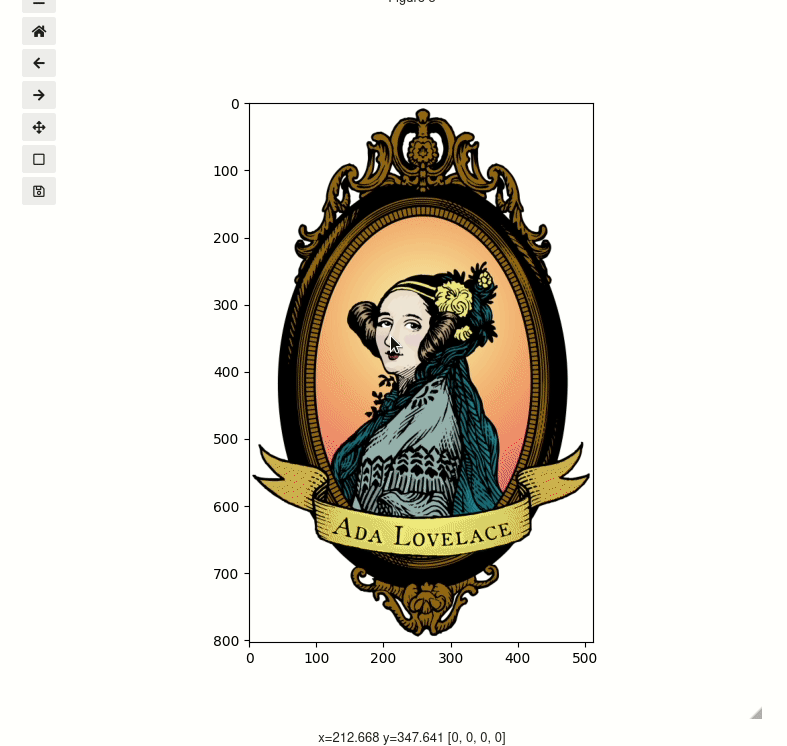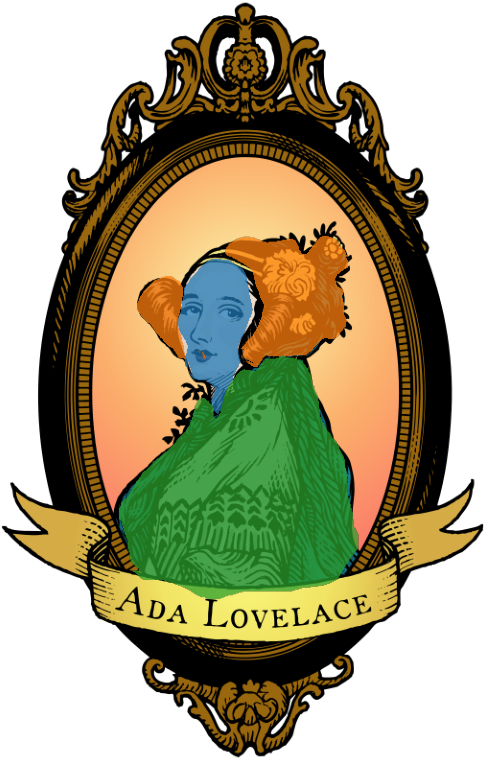# Image Segmentation#

%matplotlib ipympl
import matplotlib.cbook as cbook
import matplotlib.pyplot as plt
import numpy as np

from mpl_interactions import image_segmenter

# load a sample image
)


## Single class#

Click and drag to select regions. You can also scroll to zoom and middle click to pan.

segmenter = image_segmenter(image, mask_colors="red", mask_alpha=0.76, figsize=(7, 7))
display(segmenter)segmenter.erasing = True


### Erasing#

To start erasing instead of selecting more simply set segmenter.erasing = True. Run the following cell then try to lasso an area you have already selected

## Looking at the mask.#

The mask is transparently accessible via image_segmenter.mask. 0 is used as the value of unselected regions.

segmenter.mask

plt.figure()


## Multiple classes#

To use multiple classes set nclasses to be larger than 1. By default the tab10 color set will be used, and if that is not enough the xkcd color named colors will be used. Or you can pass a list of colors.

multi_class_segmenter = image_segmenter(image, nclasses=3, mask_alpha=0.76)
display(multi_class_segmenter)


### Change the class by changing the current_class variable#

run the below cell and then try selecting again

multi_class_segmenter.current_class = 3

plt.figure()


### Integration with ipywidgets#

To reduce friction in the labelling workflow you can construct controls for the currently selected class and whether to be in erasing mode using ipywidgets.

import ipywidgets as widgets

N_classes = 3
class_selector = widgets.Dropdown(options=list(range(1, N_classes + 1)), description="class")

def update(change):
multi_class_segmenter.current_class = class_selector.value
multi_class_segmenter.erasing = erasing_button.value

erasing_button = widgets.Checkbox(value=False, description="Erasing")
erasing_button.observe(update, names="value")

class_selector.observe(update, names="value")
multi_class_segmenter = image_segmenter(image, nclasses=3, mask_alpha=0.76)
display(widgets.HBox([erasing_button, class_selector]))
display(multi_class_segmenter)


### pre-seed a mask#

You can also load an existing mask. You will need to ensure that it does not have values greater than nclasses and that it has the same shape as the image. There are currently no safegaurds for this and when there are exceptions in a matplotlib callback they can be hard to see in the notebook - so be careful!

If you run the below cell the image should show up with the premade mask already applied!

mask = np.load("ada-mask.npy")## Styling#

### imshow parameters#

You can modify the display of the image using any kwargs accepted the imshow command. For example if we convert our example image to gray-scale then we can choose the colormap with the cmap argument.

from ipywidgets import HBox

grayscale_image = image.mean(axis=-1)
gray = image_segmenter(grayscale_image, nclasses=3, mask=mask, figsize=(5, 5), cmap="gray")
display(gray)
plasma = image_segmenter(grayscale_image, nclasses=3, mask=mask, figsize=(5, 5), cmap="plasma")
display(plasma)


## LassoSelector line#

You can change the appearance of the LassoSelector line using the lineprops kwarg. So to make the line very thick and red:

lineprops = {"color": "red", "linewidth": 10}
gray = image_segmenter(
grayscale_image, nclasses=3, mask=mask, figsize=(5, 5), cmap="gray", lineprops=lineprops
)
display(gray)